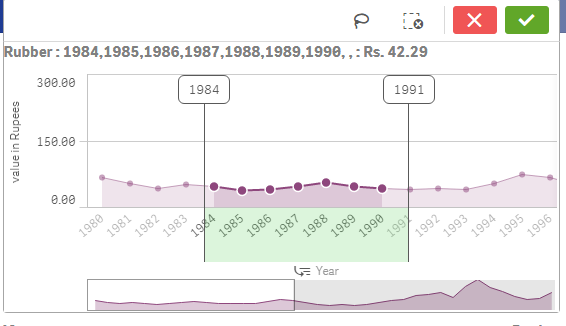# New to Qlik Sense

Discussion board where members can get started with Qlik Sense.

Announcements
Coming your way, the Qlik Data Revolution Virtual Summit. October 27-29. REGISTER
cancel
Showing results for
Did you mean:
HighlightedContributor III

## how to find the difference between measures of two selected bars in barchart?

example:

i want to find difference as

value in rupees in-

1984 = 43.44

1991 = 39.22

difference between them = 43.44 - 39.22 = 4.2216 Replies
HighlightedPartner

as a title or sub-title:

='difference btwn '&max(Year)& ' and '& min(Year)&' ='& (sum({<Year={"\$(=max(Year))"}>}Expression1) - sum({<Year={"\$(=min(Year))"}>}Expression1))

result:HighlightedContributor III

='difference btwn '&max(Year)& ' and '& min(Year)&' ='

& (sum({<Year={"\$(=max(Year))"}>}avg(Wheat2)) - sum({<Year={"\$(=min(Year))"}>}avg(Wheat2)))

so this is my query after your suggestion but i want the average here in expression but its not allowing nested aggregation here.. please help

HighlightedCreator II

Just calculate max(year) and min(year) and write expression like this.

sum({<Year={"\$(=max(Year))"}>}[value in rupees]) - sum({<Year={"\$(=min(Year))"}>}[value in rupees])

HighlightedContributor III

value in rupees is = avg(wheat2)

HighlightedCreator II

Create new field in load script using avg(wheat2) as "Value in rupees" and use the same in formula as above mentioned.

HighlightedPartner

maybe

='difference btwn '&max(Year)& ' and '& min(Year)&' ='

& (avg({<Year={"\$(=max(Year))"}>}(Wheat2)) - avg({<Year={"\$(=min(Year))"}>}(Wheat2)))

HighlightedContributor III

sorry but i forgot to say, i'm using alternate states in this charts so if u have any way of doing that using alternate states..??

HighlightedPartner

what do you mean?

HighlightedContributor III

check the qvf i've uploaded to understand the query..